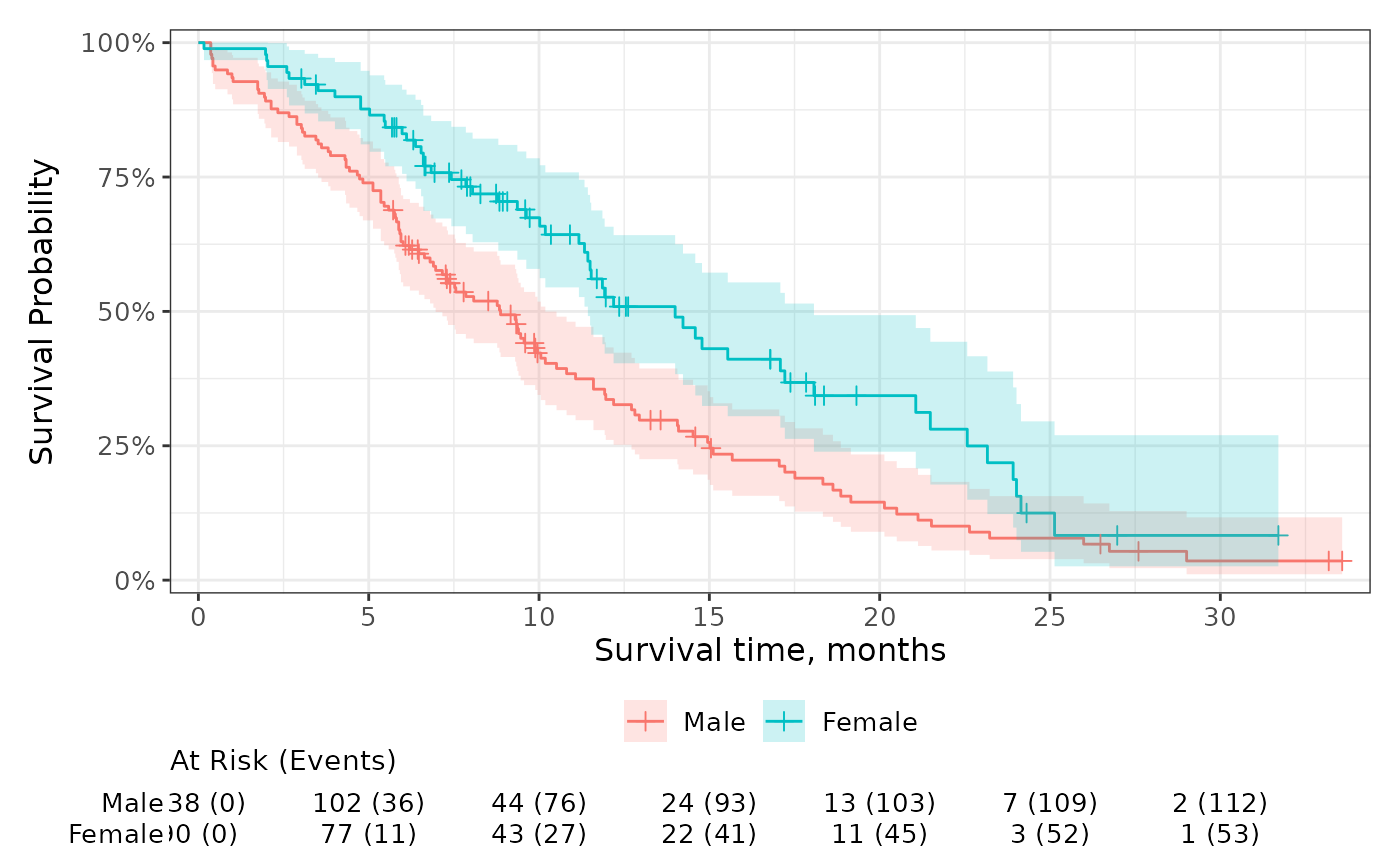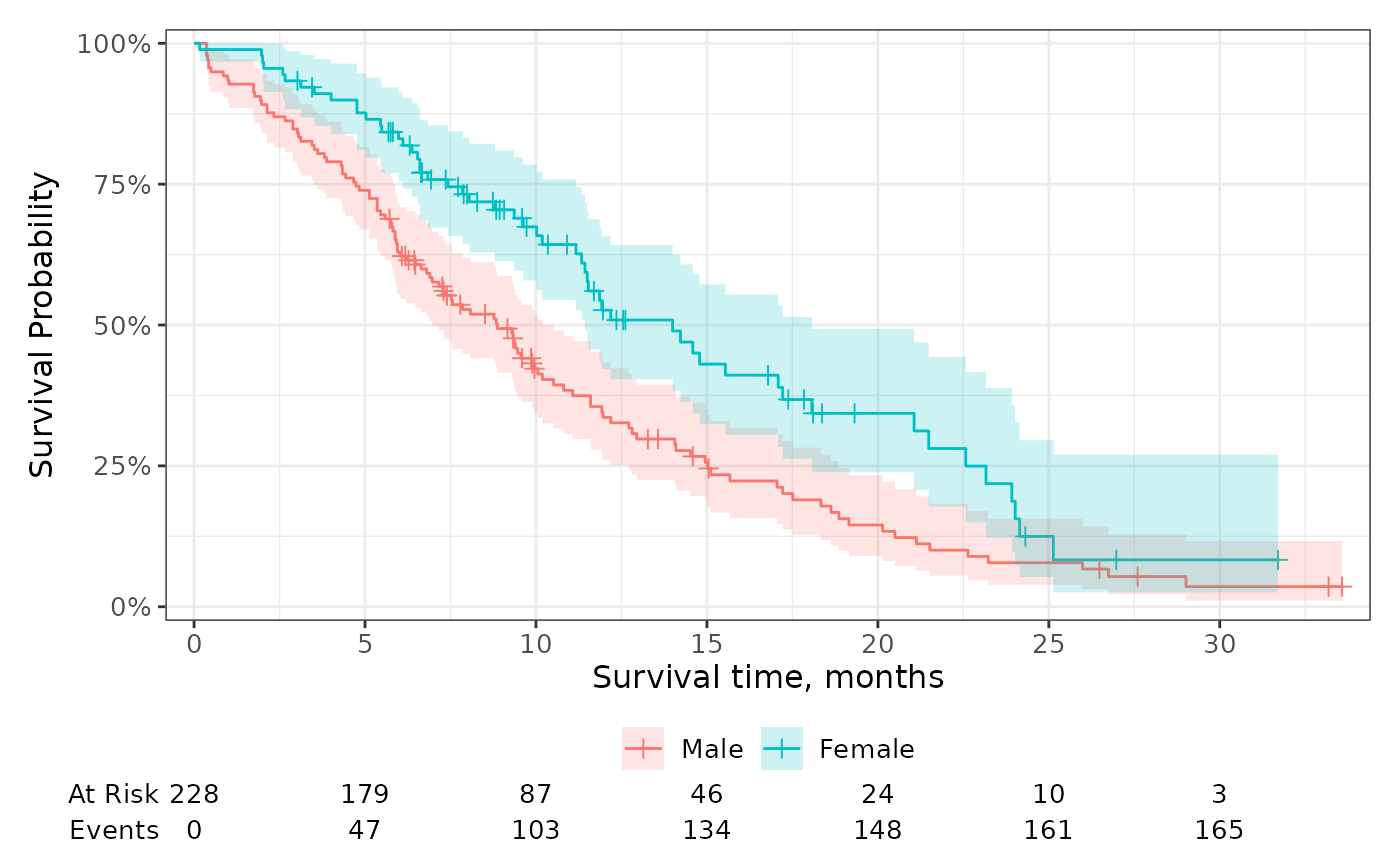Add risk tables below the plot showing the number at risk, events observed, and number of censored observations.

## Usage

add_risktable(
times = NULL,
risktable_stats = c("n.risk", "cum.event"),
risktable_group = c("auto", "strata", "risktable_stats"),
risktable_height = NULL,
stats_label = NULL,
combine_groups = FALSE,
theme = theme_risktable_default(),
size = 3.5,
...
)

## Arguments

times

numeric vector of times where risk table values will be placed. Default are the times shown on the x-axis. The times passed here will not modify the tick marks shown on the figure. To modify which tick marks are shown, use ggplot2::scale_x_continuous(breaks=).

risktable_stats

character vector of statistics to show in the risk table. Must be one or more of c("n.risk", "cum.event", "cum.censor", "n.event", "n.censor"). Default is c("n.risk", "cum.event").

• "n.risk" Number of patients at risk

• "cum.event" Cumulative number of observed events

• "cum.censor" Cumulative number of censored observations

• "n.event" Number of events in time interval

• "n.censor" Number of censored observations in time interval

risktable_group

String indicating the grouping variable for the risk tables. Default is "auto" and will select "strata" or "risktable_stats" based on context.

• "strata" groups the risk tables per stratum when present.

• "risktable_stats" groups the risk tables per risktable_stats.

risktable_height

A numeric value between 0 and 1 indicates the proportion of the final plot the risk table will occupy.

stats_label

named vector or list of custom labels. Names are the statistics from risktable_stats= and the value is the custom label.

combine_groups

logical indicating whether to combine the statistics in the risk table across groups. Default is FALSE

theme

A risk table theme. Default is theme_risktable_default()

size, ...

arguments passed to ggplot2::geom_text(...). Pass arguments like, size = 4 to increase the size of the statistics presented in the table.

a ggplot2 figure

## Customize Statistics

You can customize how the statistics in the risk table are displayed by utilizing glue-like syntax in the risktable_stats argument.

For example, if you prefer to have the number at risk and the number of events on the same row, you can use risktable_stats = "{n.risk} ({cum.event})".

You can further customize the table to include the risk estimates using elements c("estimate", "conf.low", "conf.high", "std.error"). When using these elements, you'll likely need to include a function to round the estimates and multiply them by 100.

add_risktable(
risktable_stats =
c("{n.risk} ({cum.event})",
"{round(estimate*100)}% ({round(conf.low*100)}, {round(conf.high*100)})"),
stats_label = c("At Risk (Cum. Events)", "Survival (95% CI)")
)

## Formatting Numbers

You can also pass glue-like syntax to risktable_stats to format the numbers displayed in the risk table. This is particularly helpful when working with weighted survfit2 objects for which the risk table may display too many decimals by default e.g., for weighted patients at risk.

add_risktable(
risktable_stats = c("{format(round(n.risk, 2), nsmall = 2)}",
"{format(round(n.event, 2), nsmall = 2)}"),
stats_label = c("N effective patients at risk",
"N effective events")
)

## Competing Risks

The ggcuminc() can plot multiple competing events. The "cum.event" and "n.event" statistics are the sum of all events across outcomes shown on the plot.

Visit the gallery for examples modifying the default figures

## Examples

p <-
survfit2(Surv(time, status) ~ sex, data = df_lung) %>%
ggsurvfit() +
scale_ggsurvfit()

# using the function defaults# change the statistics shown and the label
p +
risktable_stats = "n.risk",
stats_label = list(n.risk = "Number at Risk"),
)p +